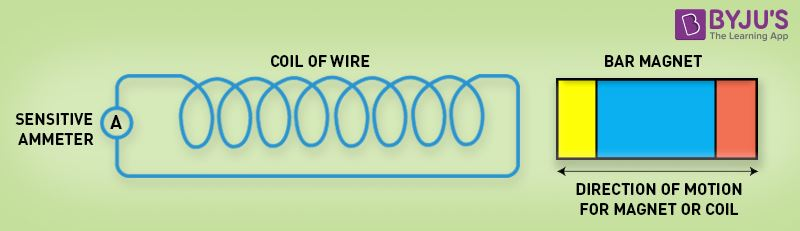# State faraday law of electro magnetic induction?

There are two laws in Faraday’s Laws of Electromagnetic Induction. The first law defines emf induction in a conductor, while the second law quantifies the emf produced.

## Faraday’s First Law of Electromagnetic Induction

Whenever a conductor is placed in a varying magnetic field, an electromotive force is induced. If the conductor circuit is closed, a current is induced which is called induced current.

### Changing the Magnetic Field Intensity in a Closed LoopMentioned here are a few ways to change the magnetic field intensity in a closed loop:

• By rotating the coil relative to the magnet.
• By moving the coil into or out of the magnetic field.
• By changing the area of a coil placed in the magnetic field.
• By moving a magnet towards or away from the coil.

## Faraday’s Second Law of Electromagnetic Induction

Faraday’s second law of electromagnetic induction states that the induced emf in a coil is equal to the rate of change of flux linkage.

The flux is the product of the number of turns in the coil and the flux associated with the coil. The formula of Faraday’s law is given below:

$\varepsilon =-N\frac{\Delta \phi }{\Delta t}$(1)(0)